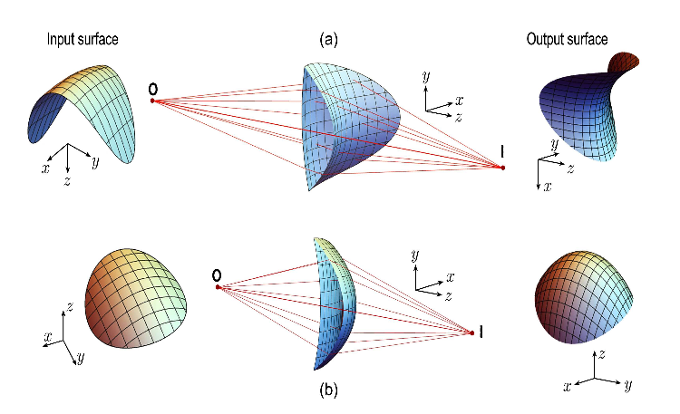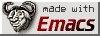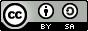# A freeform singlet lens design free of sphericalaberrationAn analytical closed-form formula for the design of freeform lenses free of spherical aberration is presented.Given the equation of the freeform input surface, the formula gives the equation of the second surface in orderto correct the spherical aberration. The derivation is based on the formal application of the variational Fermatprinciple under the standard geometrical optics approximation.